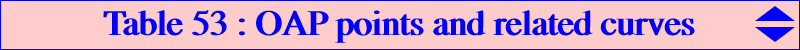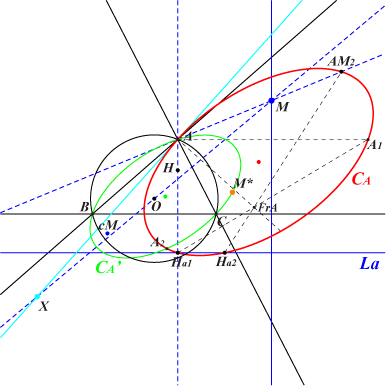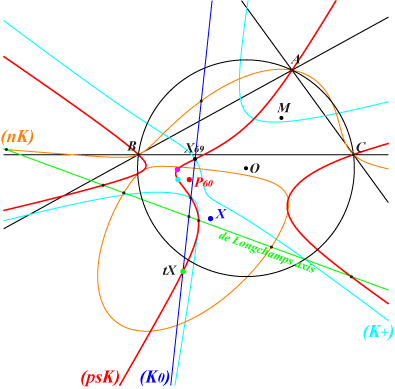Let M = u : v : w be a fixed point not lying on the sidelines of ABC, on the line at infinity, on the circumcircle (O) of ABC. P is said to be a M-OrthoAntiPedal point (or M-OAP point) if and only if M is the orthocenter of the antipedal triangle ApBpCp of P. For example, X(1) is a X(1)-OAP point since the antipedal triangle of X(1) is the excentral triangle whose orthocenter is X(1) itself. This is obviously also true for the excenters of ABC. Notations used in the sequel : J1, J2 are the circular points at infinity, HaHbHc is the orthic triangle, X is the reflection of M about O = X(3). *** A pencil of quintics and a related pK Let Hp be the orthocenter of ApBpCp, a point with relatively complicated coordinates of global degree 5 in u, v ,w. Hp and M coincide if and only if P lies on three quintics QA, QB, QC of a same pencil since u QA + v QB + w QC identically vanishes for any M. QA is a bicircular circum-quintic with nodes A, B, C, J1, J2. It also contains Ha, Hb, Hc, the infinite point of the line AM. It is then clear that any quintic of the form QS = f QA + g QB + h QC contains the M-OAP points where f : g : h are the coordinates of a point S ≠ M. All these quintics have 25 common points namely A, B, C (all quadruple), J1, J2 (all triple), Ha, Hb, Hc, hence there are four remaining common points P1, P2, P3, P4 not necessarily all real. Taking S = X, we obtain the quintic QX which splits into the line at infinity, the circumcircle (O) and the pivotal cubic pKM = pK(H x X, H) which contains the four M-OAP points. pKM is a member of CL019. *** A pencil of circular cubics Suppose that P is a M-OAP point. The lines MAp and BpCp must be perpendicular, also BpCp and AP. It follows that MAp and AP must be parallel which occurs if and only if P lies on a circular circum-cubic KA. KB and KC are defined similarly and these three cubics are in a same pencil having 9 common points namely A, B, C, J1, J2 and the four M-OAP points.Properties of KA Recall that KA is a circular circum-cubic. It also contains : • Ha, • the infinite point of the altitude AH and the real asymptote is the perpendicular at X to the sideline BC, • Oa, the A-vertex of the circumcevian triangle of X. Moreover, the tangent at A passes through X and the singular focus FA is the midpoint of AM. More generally, the singular focus of any cubic of the pencil lies on the circle with radius R/2 whose center is the midpoint of OM.*** A pencil of conics KA and pKM meet at 9 identified points namely A (with same tangent passing through X), B, C, Ha and P1, P2, P3, P4. They generate a pencil of cubics which contains a cubic decomposed into the sideline BC (passing through B, C, Ha) and a conic CA passing through the 6 remaining points. CB and CC are defined similarly and these three conics are in a same pencil having 4 common points namely P1, P2, P3, P4.Properties of CA Recall that CA is a conic passing through A with tangent passing through X. It also contains : • the infinite points of CA', the circum-conic which is the isogonal transform of the perpendicular at M to BC hence passing through the isogonal conjugate M* of M and the antipode of A on (O). • Ha1, Ha2 on the line La (reflection of BC about the complement cM of M) and on the altitude AH, the perpendicular at A to AM respectively. • AM2 = A, M /\ Ha1, M*, • A1 on the parallel at A to BC and on the line Ha1, FrA where FrA is the Frégier point related to A on CA. FrA is the intersection of the line Ha2, AM2 and the perpendicular at A to AX.Remark 1 : CA and CA' are homothetic hence CA is a rectangular hyperbola if and only if M lies on the perpendicular bisector of BC. Remark 2 : hence the pencil is a pencil of rectangular hyperbolas if and only if M = O in which case P1, P2, P3, P4 are the vertices of an orthocentric quadrilateral. See X3-OAP points below for further details. Remark 3 : if M ≠ O, the pencil contains one and only one rectangular hyperbola CH which is homothetic to the isogonal transform of the line OM. When M lies on the Euler line, it is homothetic to the Jerabek hyperbola and passes through X and cM also on the Euler line. *** A net of cubics Any decomposed cubic into a sideline of ABC and the corresponding conic CA, CB, CC contains the 7 points A, B, C, P1, P2, P3, P4. It follows that any cubic of the form KS = f x CA + g y CB + h z CC, with S as above, contains these same 7 points. KS is a member of a net which contains several remarkable circum-cubics passing through the four M-OAP points. • when S lies on the de Longchamps line (the trilinear polar of X76), KS is a circular cubic of the pencil generated by KA, KB, KC as already said above. If M is an in/excenter of ABC, all the circular cubics KS of the pencil pass through this in/excenter. If M is not an in/excenter of ABC, the pencil contains one and only one cubic passing through M. This cubic is a K0 if and only M lies on the quartic Q115. • the net always contains one equilateral cubic with asymptotes parallel to those of the McCay cubic K003. It becomes a stelloid when M lies on the Euler line. • with S = X(69), KS is a central cubic with center cM, passing through X(4), X, the infinite points of pK(X6, M). • when S is the isotomic conjugate tX of X, KS is pKM = pK(H x X, H) already mentioned. • KS is a psK when S lies on a complicated pK passing through tX. In this case, the pseudo-isopivot of KS lies on pK(X6 x ctM, X). • KS is a nK when S lies on a complicated nK whose pole is the pole of the pK above and whose root is the X(69)-isoconjugate of M.• KS is a K0 when S lies on a line passing through tX. From all this, we find that the net contains at most 3 pKs (one of them is pKM), at most 3 nK0s (very often complicated), one circular nK0. • KS is a K+ (with concurring asymptotes) when S lies on a cubic passing through X(69). *** The figure opposite represents these different loci when M = X(1). For example, (nK) is the locus of S such that KS is a nK. P60 is the only point such that KS is equilateral.*** Other curves passing through the M-OAP points • Q4 = x KA + y KB + z KC is a circular circum-quartic passing through X(2), X(3413), X(3414), the points of pK(X, X2) on (O). • Q5 = y z KA + z x KB + x y KC is a circular circum-quintic passing through X(2), Ha, Hb, Hc, the infinite points of K002. • With S = f : g : h on the line at infinity, Q'5 = f y z KA + g z x KB + h x y KC is a bicircular circum-quintic passing through S, Ha, Hb, Hc.A selection of remarkable M-OAP points and curvesX1-OAP points Apart X(1) itself, there are three X1-OAP points P1, P2, P3. They are the common points of a circle (C) and a rectangular hyperbola (H) described in the page K806. (K0) is the line X(69), X(189) and (psK) is K183 = pK(X76, X20 x X76), the isotomic transform of the Darboux cubic K004. Hence there are three pKs passing through these points namely K004, K033, K807, three members of a same pencil obtained with S = tX3345, X69, X309 respectively. Each other point S on (psK) corresponds to a psK with • pseudo-pole on K362 = pK(X213, X1) passing through X(1, 6, 33, 37, 42, 55, 65, 73, 2331), • pseudo-pivot on K007 = pK(X2, X69) passing through X(2, 4, 7, 8, 20, 69, 189, 253, 329, 1032, 1034, 5932, 14361), • pseudo-isopivot Q on K750 = pK(X213, X40) passing through X(19, 40, 55, 64, 65, 71, 2357, 3197, 3198, 8802, 8803). Note that the cubic KS with S on (K0) always contains X4. The following table gives a selection of cubics passing through these X1-OAP points according to the isotomic conjugate tS of S.tS cubic Xi on the cubic type remarks X3345 K004 see the page pK(X6, X20) central cubic X4 K033 X1, X4, X8, X10, X40, X65, X72, X3176 pK(X37, X8) central cubic X1 K259 X1, X3, X8, X220, X277, X3160 psK(X55, X2, X1) nodal cubic X972 K806 X1, X4, X40, X516, X972, X1155, X1156, X3160 K0 circular cubic X40 K807 X1, X4, X40, X46, X2910, X7952 pK(X2331, X4) X3 X1, X3, X65, X72, X218, X224 psK(X73, X7, X1) Q = X71 X20 X1, X20, X84 psK(?, X189, X1) Q = X3198 X64 X1, X64, X65, X72, X220, X225, X1490 psK(X42, X329, X1) Q = X2357 X84 X1, X8, X20, X84, X279 psK(X1, X69, X1) Q = X19 X1490 X1, X64, X1490, X3176 psK(?, X253, X1) Q = X3197 X1498 X1, X1498 psK(?, X1034, X1) Q = X8803 X3182 X1, X3182 psK(?, X1032, X1) Q = X8802 X3346 X1, X3182 psK(X65, X5932, X1) X3347 X1, X3176, X3183 psK(X33, X14361, X1) X74 X1, X65, X72, X74, X758, X2077, X5526, X5620 circular cubic X98 X1, X10, X98, X740, X2652 circular cubic X100 X1, X100, X522 circular cubic X1295 K825 X1, X20, X80, X84, X515, X1295, X1323, X7952 circular cubic X329 X1, X4, X40, X329 K0 X57 X1, X4, X40, X57, X277, X2550 K0 X189 X1, X4, X40, X189 K0 tX1903 X1, X4, X40 K0 ? X1, X9, X20, X65, X72, X84, X3668, X6282, X8806Remark 1 : any cubic with tS on the Jerabek hyperbola passes through X(65) and X(72). Remark 2 : any cubic with tS on the rectangular circum-hyperbola passing through X(40) is a K0 passing through X(4), X(40).X2-OAP points There are four X2-OAP points already mentioned in the page Q003. Recall they also lie on the rectangular hyperbola (H) passing through X(2), X(6), X(376), X(2574), X(2575), hence homothetic to the Jerabek hyperbola. In this case, any cubic KS is a K0 hence, for any point S on (psK), the cubic KS is actually a pK. This is the case of K243 = pK(X6, X376). Unfortunately, (psK) is a rather complicated pK which does not give other simple cubics. Other curves : K315 (a central cubic), K808 (a circular cubic), Q095 (a circular quintic).X3-OAP points There are four X3-OAP points which are the vertices of an orthocentric quadrilateral. They are the base points of a pencil of rectangular hyperbolas generated by : • (H1) passing through X3, X5, X155, X576, X2574, X2575, hence homothetic to the Jerabek hyperbola, • (H2) passing through X487, X488, X2043, X2044, X3413, X3414, hence homothetic to the Kiepert hyperbola. These four points also lie on Q098 and on any spK(P, Q) where P, Q are two points such that HQ = 3/4 HP (vectors). In particular, when these points lie on the Euler line, this cubic can be written under the form spK(P, Q) = K006 + T K026, where T is a real number or infinity. See Table 54 for related cubics. With T = 0, ∞, 1, -1, 2, 1/2 we obtain the cubics K006, K026, K405, K762, K810, K811, the latter being a circular cubic and actually a limit case for a spK. K812 = spK(X3524, X2) is another example, a cubic passing through the foci of the Steiner inellipse. See also K813, K814, K815.X4-OAP points There are four X4-OAP points which lie on the rectangular hyperbola (H) passing through X(3), X(20), X(185), X(2574), X(2575), hence homothetic to the Jerabek hyperbola. These four points also lie on K329, K443, K809, the stelloid K827, Q044 and on the circular cubics of a pencil with singular foci on the nine point circle, among them is K809 with focus X113.X5-OAP points There are four X5-OAP points which lie on a rectangular hyperbola (H) passing through X(140), X(550) and homothetic to the Jerabek hyperbola. These four points also lie on the stelloid K665, the central cubic K821 and also K822, K823.X6-OAP points There are four X6-OAP points which lie on the rectangular hyperbola (H) passing through X(2), X(69), X(3413), X(3414), the reflection of X(39) about X(141), hence homothetic to the Kiepert hyperbola. These four points also lie on the central cubic K805 and on the circular cubics of a pencil with singular foci on the circle with center X(182), radius R/2. See K819 for example. The net of circum-cubics passing through the four X6-OAP points contains : • pK(X4 x X1350, X4) passing through X(4), X(371), X(372), X(1350), a member of CL019. • psK(X39 x X183, X2, X3) passing through X(3), X(39), X(182), • psK(X2, X76 x X183, X76), • psK(X39, aX7735, X39). See also K820.X20-OAP points There are four X20-OAP points and one of them is X(4). Indeed, the antipedal triangle of X(4) is the antimedial triangle whose orthocenter is X(20). The remaining points are the images of the vertices of the orthic triangle under h(X4, 3) hence they lie on the altitudes of ABC. See K824 for further properties and also K855.X40-OAP points There are four X40-OAP points which lie on K691, K826.Other M-OAP points See CL019 for other (less interesting) points lying on cubics pKM.